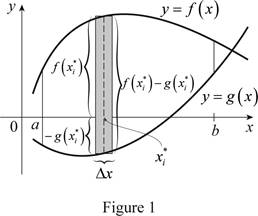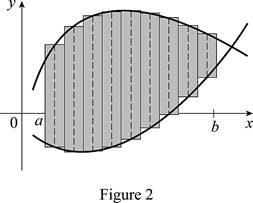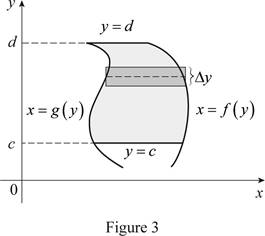# To Draw: the two typical curves y = f ( x ) and y = g ( x ) . To define: A Riemann sum that approximates the area between the two typical curves with drawing of the corresponding approximating rectangles and exact area between the two typical curves and the expression for the exact area.### Single Variable Calculus: Concepts...

4th Edition
James Stewart
Publisher: Cengage Learning
ISBN: 9781337687805### Single Variable Calculus: Concepts...

4th Edition
James Stewart
Publisher: Cengage Learning
ISBN: 9781337687805

#### Solutions

Chapter 6, Problem 1RCC

(a)

To determine

## To Draw: the two typical curves y=f(x) and y=g(x).To define: A Riemann sum that approximates the area between the two typical curves with drawing of the corresponding approximating rectangles and exact area between the two typical curves and the expression for the exact area.

Expert Solution

### Explanation of Solution

Consider the two curves y=f(x) and y=g(x).

Here, the top curve function is f(x) and the bottom curve function is g(x).

Assume f and g are continuous function and f(x)g(x) for axb.

Here, the lower limit is a and the upper limit is b.

Show the approximate ith strip rectangle with base Δx and height f(xi*)g(xi*) in the region between a and b.

Sketch the two typical curves y=f(x) and y=g(x) as shown in Figure 1.Refer to figure 1.

The two typical curves y=f(x) and y=g(x) showing the approximate ith strip rectangle is drawn.

The expression for the exact area is A=limni=1n[f(xi*)g(xi*)]Δx.

Divide the area between the two typical curves into n strips of equal width and take the entire sample points to be right endpoints, in which xi* as xi. Hence the Riemann sum is

i=1n[f(xi*)g(xi*)]Δx

Sketch thecorresponding approximating rectangles as shown in Figure 2.The better and better approximation occurs in n. Hencethe exact areaA, between the two typical curves is the sum of the areas of the corresponding approximating rectangles as shown below.

A=limni=1n[f(xi*)g(xi*)]Δx

Thus, the Riemann sum with the sketch of corresponding approximating rectangles and the exact area between the two typical curves shown.

Therefore, the approximation of the area between the two typical curves using Riemann sum with the sketch of the corresponding approximating rectangles and the sum of the areas corresponding approximating rectangles is the exact area.

(b)

To determine

Expert Solution

### Explanation of Solution

Consider the two curves x=f(y) and x=g(y).

Here, the right curve function is f(y) and the left curve function is g(y).

Assume f and g are continuous function and f(y)g(y) for cyd.

Here, the bottom limit is c and the top limit is d.

Sketch the two typical curves x=f(y) and x=g(y) is shown in Figure 3.Thus, the two typical curves y=f(x) and y=g(x) are drawn.

Normally the height calculated from the top function minus bottom one and integrating from left to right. Instead of normal calculation, use “right minus left” and integrating from bottom to top. Therefore the exact area, A written as

A=cd[f(y)g(y)]dy

Therefore, the changes of the situation if the curves have equations x=f(y) and x=g(y) is explained.

### Want to see more full solutions like this?

Subscribe now to access step-by-step solutions to millions of textbook problems written by subject matter experts!

### Have a homework question?

Subscribe to bartleby learn! Ask subject matter experts 30 homework questions each month. Plus, you’ll have access to millions of step-by-step textbook answers!